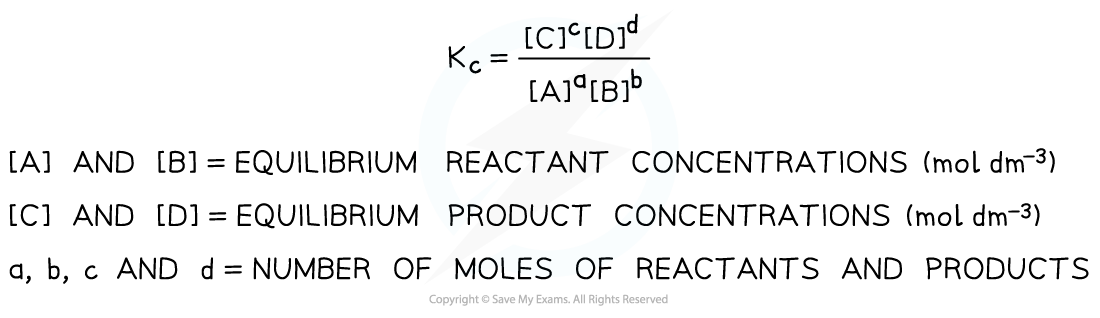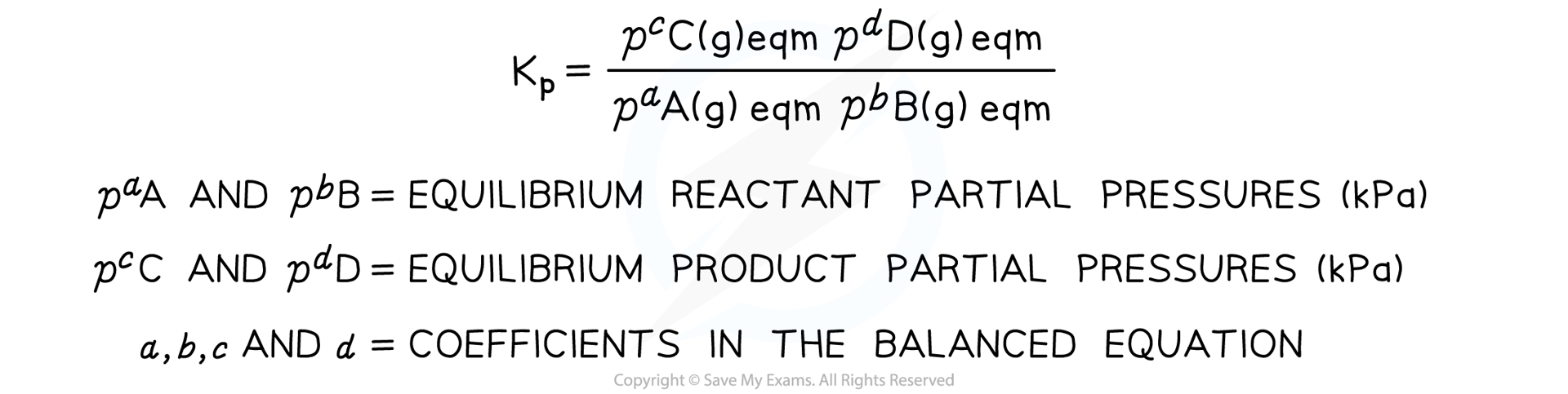# AQA A Level Chemistry复习笔记5.3.1 Kp Expressions

### Kp Expressions

• We have seen previously that equilibrium reactions can be quantified by reference to an equilibrium expression and equilibrium constant
• The equilibrium expression links the equilibrium constant, Kc, to the concentrations of reactants and products at equilibrium taking the stoichiometry of the equation into account
• So, for a given reaction:

aA + bB ⇌ cC + dD

Kc is defined as follows:Equilibrium expression linking the equilibrium concentration of reactants and products at equilibrium

• Solids are ignored in equilibrium expressions
• The Kc of a reaction is constant and only changes if the temperature of the reaction changes

#### Gaseous Equilibria

• This section covers how we manage gases in calculating the equilibrium constant and how an equilibrium yield is affected by the partial pressures of reactants and products
• Many industrial process involve reactions between gases so this application has important consequences for controlling reaction conditions
• In the generic example above, if all the substances are gases, we can show the equation with that state symbol

aA (g) + bB (g) ⇌ cC (g) + dD (g)

• We can write a different equilibrium expression in terms of the partial pressure of the gases
• This equilibrium constant is called Kp and is defined as followsEquilibrium expression linking the partial pressures of reactants and products at equilibrium

• Solids and liquids are ignored in Kp equilibrium expressions
• The Kp of a reaction is constant and only changes if the temperature of the reaction changes

#### Worked Example

Write a Kp expression for the following equilibria and deduce the units of Kp :

1. N2 (g) + 3H2 (g) ⇌ 2NH3 (g)
2. 2SO2 (g) + O2 (g) ⇌ 2SO3 (g)